#DAY 2
1
Modern Web

## JavaScript 之旅 (2)：Array.prototype.includes()「JavaScript 之旅」系列文章發文於：

# 過去的 `Array.prototype.indexOf()`

``````let array = ['JS', 'ECMA', 'TC39'];
let searchElement = 'ECMA';

console.log(array.indexOf(searchElement) !== -1);  // true
console.log(array.indexOf(searchElement) >= 0);    // true

if (~array.indexOf(searchElement)) {
console.log('找到啦！');  // 找到啦！
} else {
console.log('找不到 QQ');
}
``````

• 回傳 `-1` 代表陣列內沒有該元素
• 否則就是找到的元素的 index 值

• 不直覺：需透過 `Array.prototype.indexOf()` 來問該元素在陣列中第一次被找到的 index 為何，然後使用比較運算子 (即 `===``>=` 等) 或位元運算子 (即 `~` ) 來確定該元素是否存在
• 寫法不統一：每個人對於比較運算子的使用習慣不同，有些人會用 `!== -1`，有些人會用 `>= 0` ... 等，但明明都是一樣的行為
• 不適用於 `NaN`：因為 `Array.prototype.indexOf()` 是用嚴格相等來比較，而 `NaN !== NaN` ( `NaN` 本來就不等於自己)，所以你無法在陣列中找出 `NaN`
``````console.log([NaN].indexOf(NaN) !== -1);  // false
``````

# 現代的 `Array.prototype.includes()`

``````let array = ['JS', 'ECMA', 'TC39'];
let searchElement = 'ECMA';

console.log(array.includes(searchElement));  // true
``````

``````console.log([NaN].includes(NaN));  // true
``````

``````Array.prototype.indexOf(searchElement[, fromIndex])
Array.prototype.includes(searchElement[, fromIndex])
``````

``````let array = ['JS', 'ECMA', 'TC39', 'JS', 'ECMA'];
let searchElement = 'JS';

console.log(array.indexOf(searchElement, 2) !== -1);  // true
console.log(array.includes(searchElement, 2));  // true
``````

# spec 定義

## `Array.prototype.indexOf()` 的 spec 定義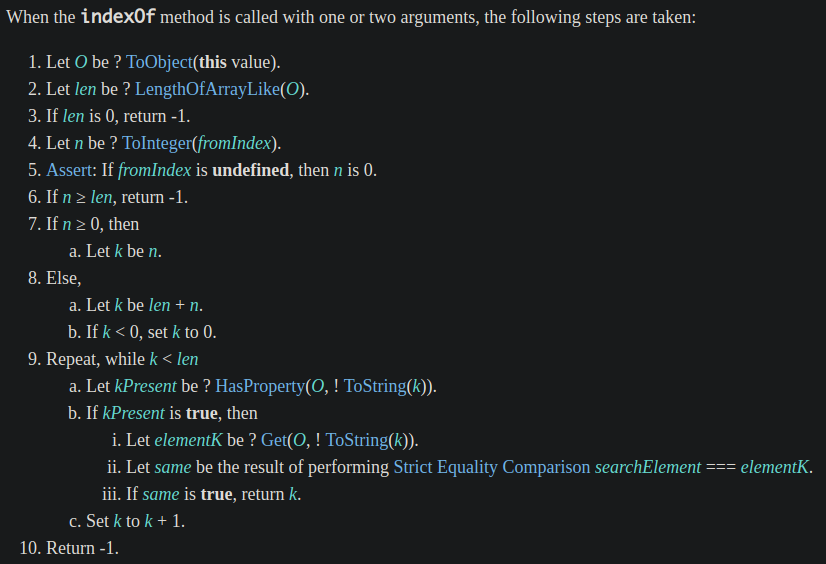## `Array.prototype.includes()` 的 spec 定義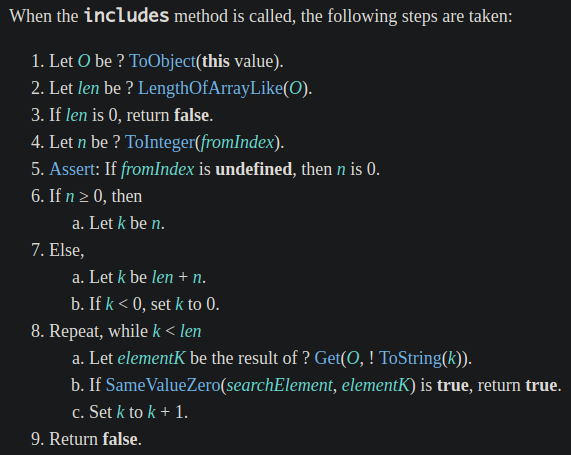• 回傳值變成 Boolean 值，而不是 index 值 (型別為 Number)
• 在比對 `searchElement` 是否 match 陣列中的元素時，使用的是 SameValueZero 演算法，而不是嚴格相等比較
• 透過 SameValueZero 演算法可以找出陣列中的 `NaN` 元素
• 不會跳過空缺的陣列元素 (即 empty slot)，而是視為 `undefined` (所以 `Array.prototype.indexOf()` 會跳過 empty slot，因為它在 spec 定義的步驟 9.a，只要沒有該 property 就會直接跳過，繼續比對下一個元素)
``````let array = Array(3);

console.log(array);                   // [empty × 3]

console.log(array.indexOf() !== -1);  // false
console.log(array.includes());        // true

console.log(array.indexOf(undefined) !== -1);  // false
console.log(array.includes(undefined));        // true
``````

## 嚴格相等比較 vs. SameValueZero

### 嚴格相等比較 的 spec 定義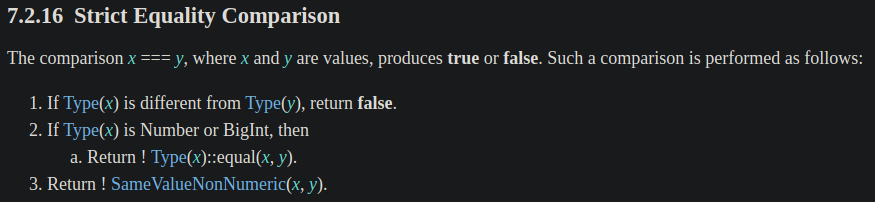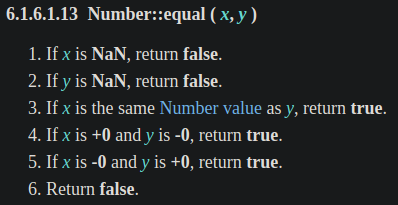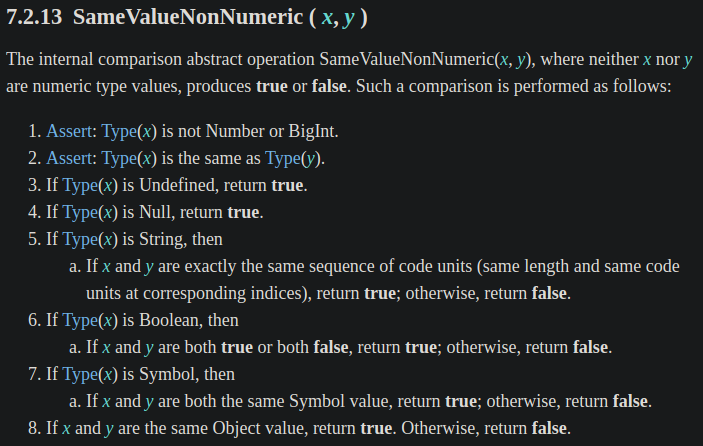• 值為 `null``undefined` 時會回傳 `true`
• `String` 型別就比對字串
• `Boolean``Symbol``Object` 型別都是比較值是否相同 (但 Object 型別的值必須是同一個參考)

``````let array = [{id: 1}, {id: 2}, {id: 3}];

console.log(array.indexOf({id: 2}) !== -1);  // false
console.log(array.includes({id: 2}));        // false
``````

``````let item = {id: 2};
let array = [{id: 1}, item, {id: 3}];

console.log(array.indexOf(item) !== -1);  // true
console.log(array.includes(item));        // true
``````

### SameValueZero 的 spec 定義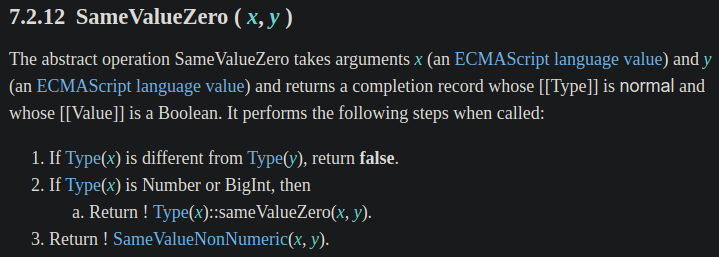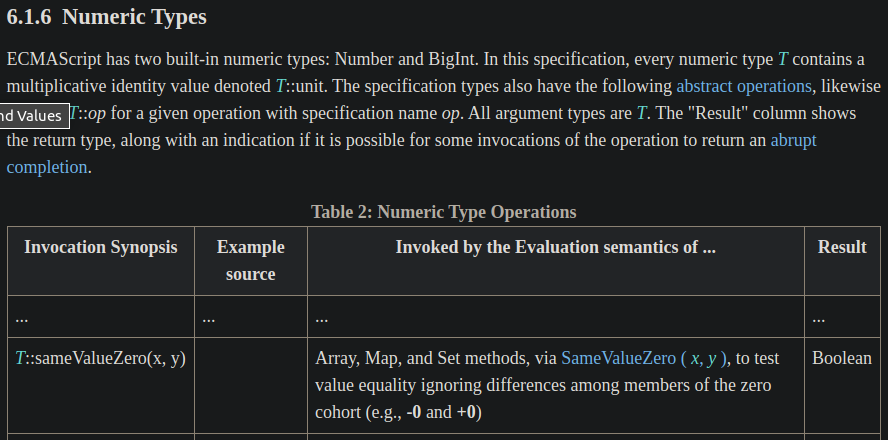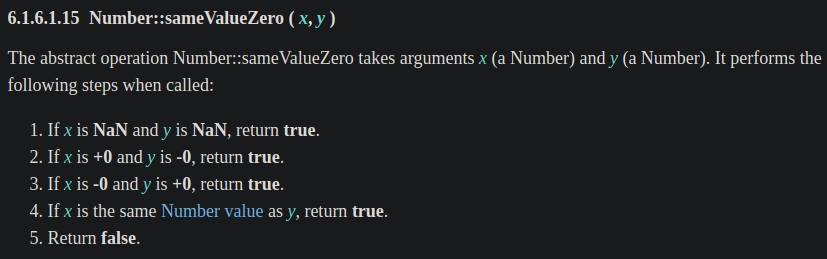# polyfill

``````// ref: https://developer.mozilla.org/zh-TW/docs/Web/JavaScript/Reference/Global_Objects/Array/includes#Polyfill
if (!Array.prototype.includes) {
Object.defineProperty(Array.prototype, 'includes', {
value: function(searchElement, fromIndex) {

if (this == null) {
throw new TypeError('"this" is null or not defined');
}

// 1. Let O be ? ToObject(this value).
var o = Object(this);

// 2. Let len be ? LengthOfArrayLike(O)
//    即 Let len be ? ToLength(? Get(O, "length")).
var len = o.length >>> 0;

// 3. If len is 0, return false.
if (len === 0) return false;

// 4. Let n be ? ToInteger(fromIndex).
// 5. Assert: If fromIndex is undefined, this n is 0.
var n = fromIndex | 0;

// 6. If n >= 0, then
//   a. Let k be n.
// 7. Else n < 0,
//   a. Let k be len + n.
//   b. If k < 0, set k to 0.
var k = Math.max(n >= 0 ? n : len - Math.abs(n), 0);

function sameValueZero(x, y) {
return (
x === y || (
typeof x === 'number' && typeof y === 'number' &&
isNaN(x) && isNaN(y)
)
);
}

// 8. Repeat, while k < len
while (k < len) {
// a. Let elementK be the result of ? Get(O, ! ToString(k)).
// b. If SameValueZero(searchElement, elementK) is true, return true.
if (sameValueZero(o[k], searchElement)) return true;
// c. Set k to k + 1.
k++;
}

// 9. Return false
return false;
}
});
}
``````

# 資料來源：

JavaScript 之旅30